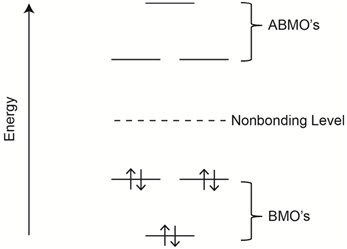# 2.3: Effect of Non-bonding Electrons

•• Thomas Wenzel
• Bates College
$$\newcommand{\vecs}{\overset { \rightharpoonup} {\mathbf{#1}} }$$ $$\newcommand{\vecd}{\overset{-\!-\!\rightharpoonup}{\vphantom{a}\smash {#1}}}$$$$\newcommand{\id}{\mathrm{id}}$$ $$\newcommand{\Span}{\mathrm{span}}$$ $$\newcommand{\kernel}{\mathrm{null}\,}$$ $$\newcommand{\range}{\mathrm{range}\,}$$ $$\newcommand{\RealPart}{\mathrm{Re}}$$ $$\newcommand{\ImaginaryPart}{\mathrm{Im}}$$ $$\newcommand{\Argument}{\mathrm{Arg}}$$ $$\newcommand{\norm}{\| #1 \|}$$ $$\newcommand{\inner}{\langle #1, #2 \rangle}$$ $$\newcommand{\Span}{\mathrm{span}}$$ $$\newcommand{\id}{\mathrm{id}}$$ $$\newcommand{\Span}{\mathrm{span}}$$ $$\newcommand{\kernel}{\mathrm{null}\,}$$ $$\newcommand{\range}{\mathrm{range}\,}$$ $$\newcommand{\RealPart}{\mathrm{Re}}$$ $$\newcommand{\ImaginaryPart}{\mathrm{Im}}$$ $$\newcommand{\Argument}{\mathrm{Arg}}$$ $$\newcommand{\norm}{\| #1 \|}$$ $$\newcommand{\inner}{\langle #1, #2 \rangle}$$ $$\newcommand{\Span}{\mathrm{span}}$$$$\newcommand{\AA}{\unicode[.8,0]{x212B}}$$

## Compare the UV absorption spectrum of benzene and pyridine.

Benzene has a set of conjugated $$\pi$$-bonds and the lowest energy transition would be a $$\pi$$-$$\pi$$* transition as shown in Figure $$\PageIndex{17}$$.Figure $$\PageIndex{17}$$. Representation of the lowest energy transition in benzene.

The UV/VIS absorption spectrum for benzene is shown in Figure $$\PageIndex{18}$$.Figure $$\PageIndex{18}$$. Ultraviolet absorption spectrum of benzene.

Benzene absorbs radiation in the vacuum ultraviolet over the range from 160-208 nm with a $$\lambda$$max value of about 178 nm. Pyridine has a similar conjugation of double bonds comparable to what occurs in benzene.Figure $$\PageIndex{19}$$ shows filled bonding molecular orbitals (BMOs), empty anti-bonding molecular orbitals (ABMOs) and the location of non-bonding electrons.Figure $$\PageIndex{19}$$. Representation of the relative energies of bonding molecular orbitals (BMOs), anti-bonding molecular orbitals (ABMOs) and non-bonding electrons.

For pyridine, the lowest energy transition involves the n-$$\pi$$* orbitals and this will be much lower in energy than the $$\pi$$-$$\pi$$* transition in pyridine or benzene. The UV/VIS absorption spectrum of pyridine is shown in Figure $$\PageIndex{20}$$.Figure $$\PageIndex{20}$$. UV/VIS absorption spectrum of pyridine.

The shift toward higher wavelengths when compared to benzene is quite noticeable in the spectrum of pyridine, where the peaks from 320-380 nm represent the n-$$\pi$$* transition and the peak at about 240 nm is a $$\pi$$-$$\pi$$* transition. Note that intensity and therefore the molar absorptivity of the n-$$\pi$$* transition is lower than that of the $$\pi$$-$$\pi$$* transition. This is usually the case with organic compounds.

Dye molecules absorb in the visible portion of the spectrum. They absorb wavelengths complementary to the color of the dye. Most $$\pi$$-$$\pi$$* transitions in organic molecules are in the ultraviolet portion of the spectrum unless the system is highly conjugated. Visible absorption is achieved in dye molecules by having a combination of conjugation and non-bonding electrons. Azo dyes with the N=N group are quite common, one example of which is shown in Figure $$\PageIndex{21}$$.Figure $$\PageIndex{21}$$. Structure of yellow azo dye.

This page titled 2.3: Effect of Non-bonding Electrons is shared under a CC BY-NC 4.0 license and was authored, remixed, and/or curated by Thomas Wenzel via source content that was edited to the style and standards of the LibreTexts platform; a detailed edit history is available upon request.